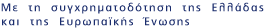Now showing items 1-2 of 2

• #### Nonstationary Extrapolated Modulus Algorithms for the solution of the Linear Complementarity Problem ﻿

(2009)
The Linear Complementarity Problem (LCP) has many applications as, e.g., in the solution of Linear and Convex Quadratic Programming, in Free Boundary Value problems of Fluid Mechanics, etc. In the present work we assume ...
• #### ON ITERATIVE SOLUTION FOR LINEAR COMPLEMENTARITY PROBLEM WITH AN H+-MATRIX ﻿

(2012)
The numerous applications of the linear complementarity problem (LCP) in, e.g., the solution of linear and convex quadratic programming, free boundary value problems of fluid mechanics, and moving boundary value problems ...